In this page we will recap through some basic information related to Geometry and then we will be solving Geometry Questions and Answers which will help you in tackling questions if asked in the exams.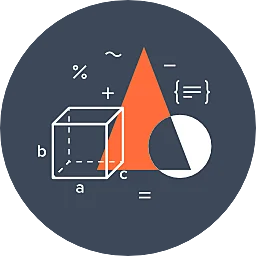Concept

Before diving into two-dimensional and three-dimensional shapes, consider the basic geometric objects that create these shapes: points, lines, line segments, rays, and planes.

• A point is represented by a dot and shows a location in space.
• A line is a set of straight points that extends forever in both directions as depicted by arrows on both ends.
• Rays are lines that end on one side. Line segments end on both sides. Planes are surfaces that extend forever in all directions.

There are two types of shapes where the concept of geometry revolves, those are 3D and 2D shapes. Let us know them about in brief:

• Two-dimensional objects only have two dimensions: length and width.
• Unlike two-dimensional objects, three-dimensional objects have a third dimension, depth, and are, therefore, not flat. Cubes, spheres, and pyramids are examples of three-dimensional objects. A cube is an object made of six square sides.

Sample Geometry Questions and Answers are given below:

1. If angles P and Q are complementary to one another, and angle P is two times of angle Q. Calculate the measures of angles P and Q.60°

60°

53.42%

90°

90°

27.4%

120°

120°

9.59%

None

None

9.59%

Let the measure of angle P be P, and the measure of angle Q be Q.

P = 2Q

Given that angles P and Q are complementary. Therefore,

P + Q = 900

But, P = 2Q. Therefore,

2Q + Q = 90

3Q = 90

Q= 90/3 = 300

P =2Q = 60°2. What is the value of angle A in the given figure?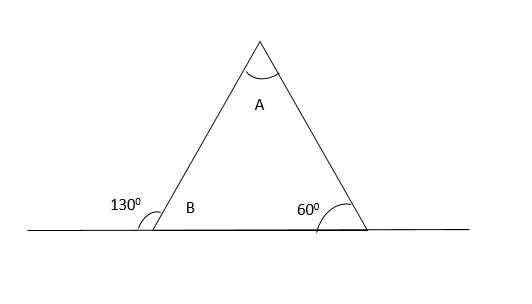30°

30°

9.59%

70°

70°

83.56%

80°

80°

2.74%

90°

90°

4.11%

To calculate the measure of angle A, we have to calculate the value of angle B.

Angle B = 180-130= 500

Therefore, the measure of angle A = 180 – (50+60)

A = 180-110

A =70°3. The given triangle ABC is a right triangle. Angle ABC is 600. Find the measure of triangle APC.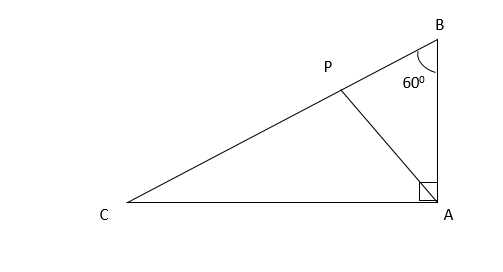56°

56°

7.14%

40°

40°

10.71%

75°

75°

66.07%

80°

80°

16.07%

The sum of all the angles in the given triangle ABC is 1800

180 = 60 + 90 + BCA

Therefore, angle BCA = 180-150= 300

Angle APC = 60 + 45- 180

180-105 = 75°4. In the given figure, angle CEB = 1200. Calculate the measure of angle AED.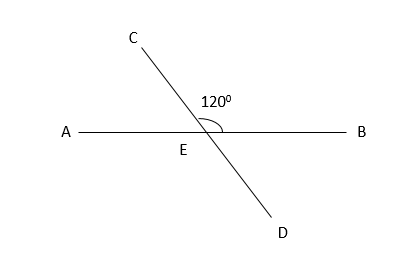56°

56°

3.08%

40°

40°

6.15%

120°

120°

87.69%

80°

80°

3.08%

From the given figure, angle DEB = 180-120 = 600

Therefore, angle AED = 180-30 = 120°5. What is the measure of angle A in the given figure?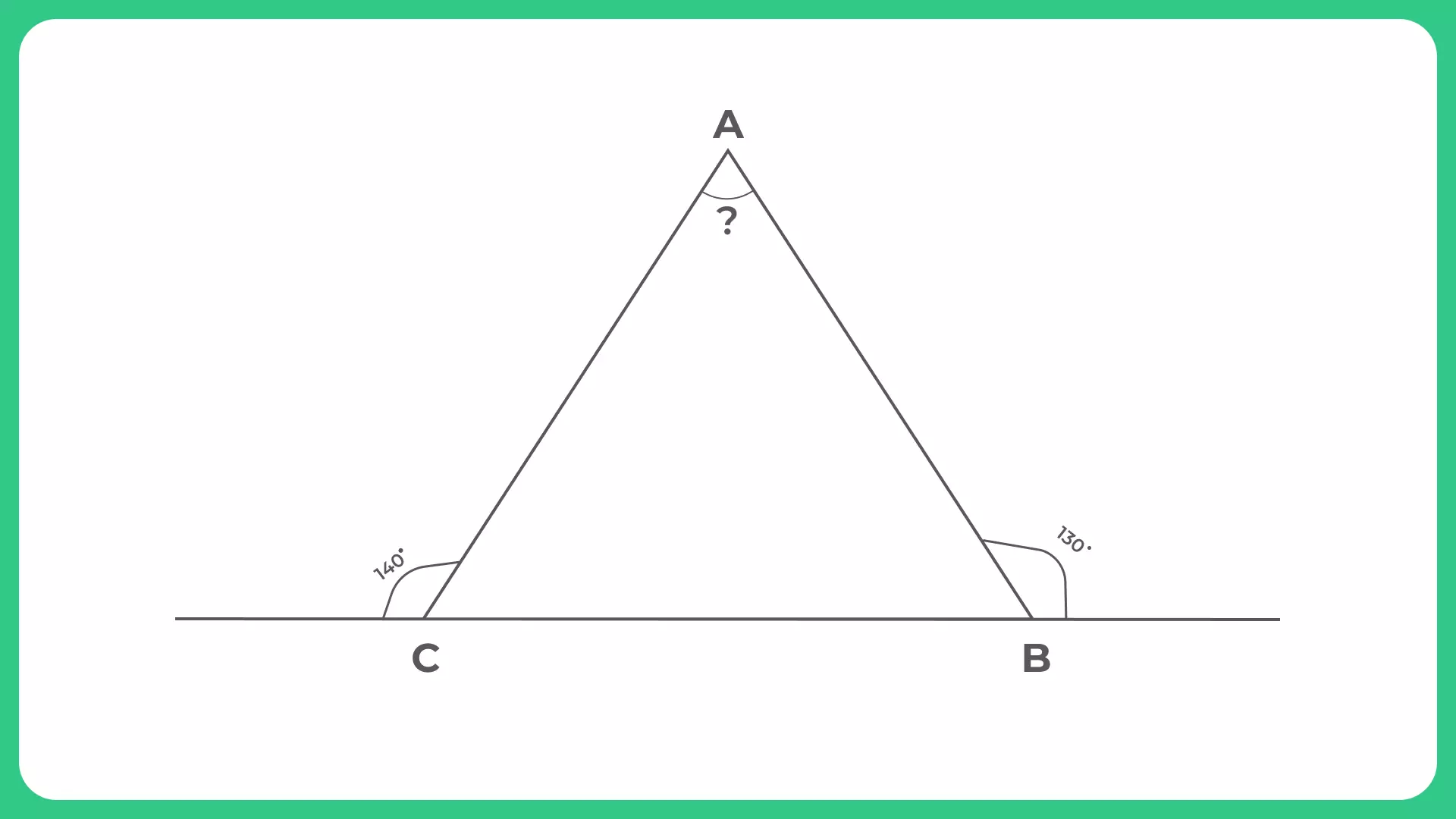66°

66°

5%

90°

90°

86.67%

85°

85°

5%

30°

30°

3.33%

The internal angle B in the given triangle = 180-130 = 500

The internal angle C in the given triangle = 180-140 = 400

Therefore, in the given triangle, measure of angle A = 180 – (40 + 50)

180 – 90 = 90°6. Calculate the value of angle EDB when the remaining angles are given.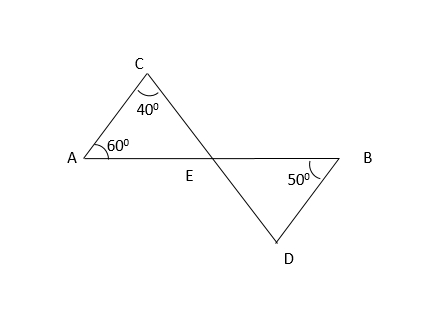50°

50°

72.13%

40°

40°

19.67%

75°

75°

4.92%

90°

90°

3.28%

In the given triangle ACE, vale of angle AEC = 180- (60+40)

= 180 – 100

=800

Also, the given angle DEB = 800 as it is directly opposite to angle AEC.

= In triangle DEB, value of angle EDB = 180 – (80+50)

=180 – 130

50°7. In the given figure, determine the value of angle RPB.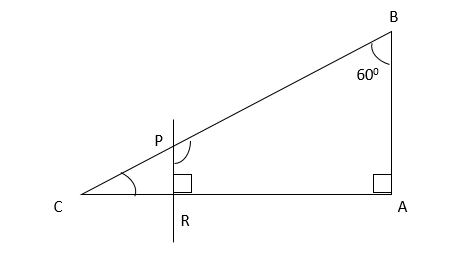60°

60°

20%

120°

120°

69.09%

80°

80°

7.27%

70°

70°

3.64%

In the given triangle ABC, value of angle C = 180 – (60+90)

180 – 150 = 300

Now, in the given triangle PCR, value of angle R = 900

Value of angle CPR = 180 – (90+30)

180 – 120 = 60°

Now, value of angle RPB = (180 - 60) = 12008. Calculate the area enclosed by ABDEF.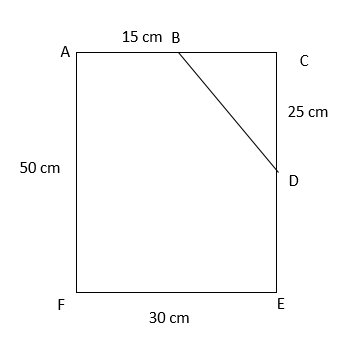1312.5 cm2

1312.5 cm2

76.6%

200 cm2

200 cm2

8.51%

350 cm2

350 cm2

2.13%

None of the above

None of the above

12.77%

Area of ABDEF = area of ACEF – area of triangle BCD

= 50 x 30 – ((1/2)*15*25)

= 50 x 30 – 187.5

= 1500 – 187.5

= 1312.5 cm29. What will be the area of the presented figure when all its sides are given?11 cm²

11 cm²

18.42%

13 cm²

13 cm²

15.79%

10.72 cm²

10.72 cm²

39.47%

None of the above

None of the above

26.32%

The presented figure can be divided into two triangles to calculate the area.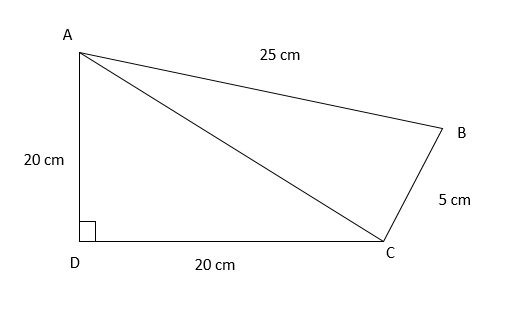We can also label the given figure to identify and mark the 2 triangles.

Now the figure consists of triangles: ADC and triangle ACB.

The area of the given triangle can be calculated when we know the measure of length AC.

For this, in the given triangle, ADC,

AC2 = 202 + 202

AC =√400+400

AC = √800

AC = 28.28

Area of triangle ADC = ½*20*20

= 200 cm²

We also need the area of the triangle ABC

Area of the triangle when its three sides are given = √p(p-a)(p-b)(p-c)

Where p = (a+b+c)/2

P = (25+5+28.28)/2 = 29.14

Therefore, area of triangle = √29.14(29.14-25)(29.14-5)(29.14-28.28)

= √115.08

= 10.72 cm²10. Determine the area of the given triangle.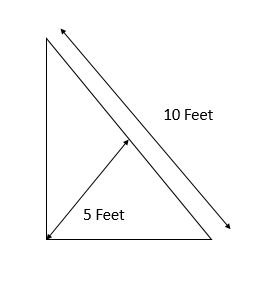25cm²

25cm²

72.34%

15cm²

15cm²

6.38%

10 cm²

10 cm²

8.51%

55cm²

55cm²

12.77%

The solution is to rotate the triangle to bring the 10 feet side at the bottom.

Therefore, the area of the triangle =

Area=

= 25cm²

&nbsp;×

### Related Banners

Get PrepInsta Prime & get Access to all 200+ courses offered by PrepInsta in One Subscription

## Get over 200+ course One Subscription

Courses like AI/ML, Cloud Computing, Ethical Hacking, C, C++, Java, Python, DSA (All Languages), Competitive Coding (All Languages), TCS, Infosys, Wipro, Amazon, DBMS, SQL and others